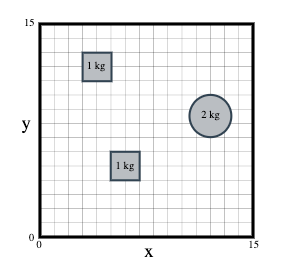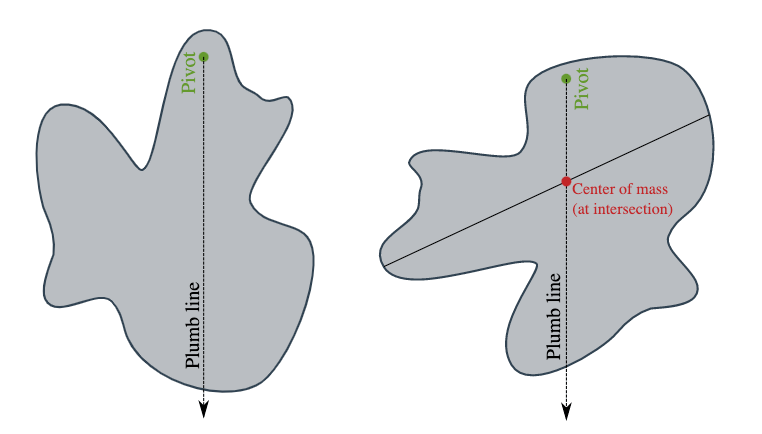MCAT Content / Force / Center Of Mass

### Center of Mass

Topic: Force

The center of mass is a position defined relative to an object or system of objects. It is the average position of all the parts of the system, weighted according to their masses.

The center of mass can be found by vector addition of the weighted position vectors which point to the center of mass of each object in a system. One quick technique which lets us avoid the use of vector arithmetic is finding the center of mass separately for components along each axis:Together, these give the full coordinates (, of the center of mass of the system.Complex objects can often be represented as collections of simple shapes, each with uniform mass. We can then represent each component shape as a point mass located at the centroid. Voids within objects can even be accounted for by representing them as shapes with negative mass.The center of gravity is the point through which the force of gravity acts on an object or system. In most mechanics problems the gravitational field is assumed to be uniform. The center of gravity is then in exactly the same position as the center of mass. The terms center of gravity and center of mass tend to often be used interchangeably since they are often at the same location.

There are a couple of useful experimental tests that can be done to determine the center of mass of rigid physical objects.

The table edge method can be used to find the center of mass of small rigid objects with at least one flat side. The object is pushed slowly without rotating along the surface of a table towards an edge. At the point where the object is just about to fall, a line is drawn parallel to the table edge. The procedure is repeated with the object rotated 90°. The intersection point of the two lines gives the location of the center of mass in the plane of the table.The plumb line method is also useful for objects which can be suspended freely about a point of rotation. An irregularly shaped piece of cardboard suspended on a pin-board is a good example of this. The cardboard pivots freely around the pin under gravity and reaches a stable point. A plumb line is hung from the pin and used to mark a line on the object. The pin is moved to another location and the procedure repeated. The center of mass then lies beneath the intersection point of the two lines.One useful application of the center of mass is determining the maximum angle that an object can be tilted before it will topple over.

The figure below shows a cross-section of a truck. The truck has been poorly loaded with many heavy items loaded on the left-hand side. The center of mass is shown as a red dot. A red line extends down from the center of mass, representing the force of gravity. Gravity acts on all the weight of the truck through this line.

If the truck is tipped at an θt, then all the weight of the truck will be supported by the left-most edge of the left wheel. Should the angle be further increased then the point of support will move outside of any point of contact with the road and the truck is guaranteed to topple over. The angle θt is the topple limit.When the term reference frame is used in physics it refers to the coordinate system used for calculations. A reference frame has a set of axes and an origin (zero point). In most problems, the reference frame is fixed relative to the laboratory and a convenient (but arbitrary) origin point is chosen. This is known as the laboratory reference frame. However, in classical physics, it is also possible to use any other reference frame and expect the laws of physics to hold within it. This includes reference frames which are moving relative to the laboratory.

One very useful property of the center of mass is that it can be used to define the origin of a moving reference frame for a system. This reference frame is sometimes called the COM frame. The COM frame is particularly useful in collision problems. It turns out that the momentum of a fully-defined system measured in the COM frame is always zero. This means that calculations can often be much simpler when done in the COM frame compared to the laboratory reference frame.

Practice Questions

Electrostatics, medicine, and metal spheres

MCAT Official Prep (AAMC)

Key Points

• The center of mass (COM) is a statement of spatial arrangement of mass (i.e. distribution of mass within the system).

• The experimental determination of the center of mass of a body uses gravity forces on the body and relies on the fact that in the parallel gravity field near the surface of the earth the center of mass is the same as the center of gravity.

• For a 2D object, an experimental method for locating the center of mass is to suspend the object from two locations and to drop plumb lines from the suspension points. The intersection of the two lines is the center of mass.

• The center of gravity is the point through which the force of gravity acts on an object or system.

• The table edge method can be used to find the center of mass of small rigid objects with at least one flat side.

• One useful application of the center of mass is determining the topple limit, the maximum angle that an object can be tilted before it will topple over.

• Another useful property of the center of mass is that it can be used to define the origin of a moving reference frame for a system.

Key Terms

Center of mass: A point representing the mean position of the matter in a body or system.

Vector addition: The operation of adding two or more vectors together into a vector sum.

Mass: The physical property of matter that depends on size and shape of matter, and is expressed as kilograms by the SI system.

Center of gravity: A point from which the weight of a body or system may be considered to act.

Table edge: A method used to find the center of mass of small rigid objects with at least one flat side.

Plumb line: A method useful for determining the center of mass for a complex planar shape with unknown dimensions. It relies on finding the center of mass of a thin body of homogeneous density having the same shape as the complex planar shape.

Topple limit (θt): The maximum angle that an object can be tilted before it will topple over.

Reference frame: A frame of reference consists of an abstract coordinate system and the set of physical reference points that uniquely fix the coordinate system and standardize measurements within that frame.

Laboratory reference frame: A frame of reference centered on the laboratory in which the experiment is done. This is the reference frame in which the laboratory is at rest.

Momentum: The quantity of motion of a moving body, measured as a product of its mass and velocity.

Billing Information
We had trouble validating your card. It's possible your card provider is preventing us from charging the card. Please contact your card provider or customer support.
{{ cardForm.errors.get('number') }}
{{ registerForm.errors.get('zip') }}
{{ registerForm.errors.get('coupon') }}
Tax: {{ taxAmount(selectedPlan) | currency spark.currencySymbol }}

Total Price Including Tax: {{ priceWithTax(selectedPlan) | currency spark.currencySymbol }} / {{ selectedPlan.interval | capitalize }}